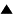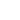# Mathematical Methods of Population Genetics

The mathematical methods of population genetics theory characterize quantitatively the gene distribution dynamics in evolving populations [1-3]. There are two types of models: deterministic and stochastic. Deterministic models are based on the approximation of an infinitely large population size. In this case the fluctuations of gene frequencies (in a gene distribution) can be neglected and the population dynamics can be described in terms of the mean gene frequencies. The stochastic models describe the probabilistic processes in finite size populations. Here we review very briefly the main equations and mathematical methods of population genetics by considering the most representative examples.

## Deterministic models

Let's consider a population of diploid1) organisms with several alleles2) A1 , A2 ,..., AK in some locus3). We assume that the organism fitness is determined mainly by the considered locus. Designating the number of organisms and the fitness of the gene pair Ai Aj by nij and Wij, respectively, we can introduce the genotype and gene frequencies Pij and Pi , as well as the mean gene fitnesses Wi in accordance with the expressions [1,2,4]:

 Pij = nij /n , Pi = S j Pij , Wi =Pi-1 S j Wij Pij , (1)

where n is the population size, index i refers to the class of organisms {Ai Aj}j=1,2,..., K , which contain the gene Ai. The population is supposed to be a panmictic4) one: during reproduction the new gene combinations are chosen randomly throughout in the whole population. For panmictic populations the Hardy-Weinberg principle can be approximately applied :

 Pij =Pi Pj , i, j = 1,...,K . (2)

Eqs. (2) implies, that during mating the genotypes are formed proportionally to the corresponding gene frequencies.

The evolutionary dynamics of the population in terms of the gene frequencies Pi can be described by the following differential equations [1,2,4]:

 dPi /dt = Wi Pi - Pi - S j uji Pi + S j uij Pj , i = 1,...,K , (3)

where t is time, <W> = S ij Wij Pij is the mean fitness in a population; uij is the mutation rate of the transition Aj --> Ai, uii =0 (i, j = 1,..., K). The first term in the right side of Eqs. (3) characterizes the selection of the organisms in accordance with their fitnesses, the second term takes into account the condition S i Pi = 1, the third and fourth terms describe the mutation transitions.

Note that similar equations are used in the quasispecies model (for the deterministic case) .

Neglecting the mutations, we can analyze the dynamics of genes in the population by means of the equations:

 dPi /dt = Wi Pi - Pi , i = 1,...,K . (4)

Using (1), (2), (4), one can deduce (under the condition that the values Wij are constant), that the rate of increase for the mean fitness is proportional to the fitness variance V = S i Pi ( Wi - <W>)2 [1,3]:

 d/dt = 2 S i Pi ( Wi - )2 . (5)

In accordance with (4), (5), the mean fitness <W> always increases, until an equilibrium state (dPi /dt = 0) is reached.

The equation (5) characterizes quantitatively The Fundamental Theorem of Natural Selection (R.A.Fisher, 1930), which in our case can be formulated as follows :

In a sufficiently large panmictic population, where the organisms' fitness is determined by one locus and the selection pressure parameters are defined by the constant values Wij, the mean fitness in a population increases, reaching a stationary value in some genetic equilibrium state. The increase rate of the mean fitness is proportional to the fitness variance; it becomes zero in an equilibrium state.

The described model is a simple example of the deterministic approach. The wide spectrum of analogous models, which describe the different particularities, concerning several gene loci, age and female/male distributions in a population, inbreeding, migrations, subdivisions of populations, were developed and investigated, especially in connection with concrete genetic data interpretations [1,3,4].

## Stochastic models

Deterministic models provide effective methods for evolving population description. However, they use the approximation of an infinitely large population size, which is too strong for many real cases. To overcome this limitation, the probabilistic methods of population genetics were developed [1,3,4,6]. These methods include the analysis by means of Markov chains (especially, by using the generating functions) [4,7], and the diffuse approximation [1,3,4,6].

Below we sketch the main equations and some examples of the diffuse approximation. This approximation provides a non-trivial and effective method of population genetics.

We consider a population of diploid organisms with two alleles A1 and A2 in a certain locus. The population size n is supposed to be finite, but sufficiently large, so that the gene frequencies can be described by continuous values. We also suppose that the population size n is constant.

Let's introduce the function j = j (X,t|P,0) , which characterizes the probability density of the frequency X of the gene A1 at the time moment t under condition that the initial frequency (at t = 0) of this gene is equal to P. Under the assumption that the changes of the gene frequencies at one generation are small, the populations dynamics can be described approximately by the following partial differential equations [1,3,4]:

 ¶j/¶t = - ¶ (Md X j )/¶X + (1/2) ¶ 2(Vd X j )/¶X2 , (6)
 ¶j/¶t = Md P ¶j/¶P + (1/2)Vd P ¶ 2j/¶P2 , (7)

where Md X , Md P and Vd X , Vd P are the mean values and the variances of the changes of the frequencies X, P during one generation; time unit is equal to one generation. Eq. (6) is the forward Kolmogorov differential equation (in physics it is called the Fokker-Planck equation); Eq. (7) is the backward Kolmogorov differential equation.

The first terms in the right sides of Eqs. (6), (7) describe a systematic selection pressure, which is due to the fitness difference of the genes A1 and A2. The second terms characterize the random drift of the frequencies, which is due to the fluctuations in the finite size population.

Using Eq. (6), one can determine the time evolution of the gene frequency distribution, Eq. (7) provides the means to estimate the probabilities of gene fixation.

Assuming that 1) the fitnesses of gene A1 and A2 are equal to 1 and 1-s, respectively and 2) the gene contributions to the fitnesses of the gene pairs A1 A1, A1 A2, and A2 A2 are additive, one can obtain, that the values Md X , Md P and Vd X , Vd P are determined by the following expressions [1,3,4]:

 Md X = sX(1-X) , Md P = sP(1-P) , Vd X = X(1-X)/2n , Vd P = P(1-P)/2n . (8)

If the evolution is purely neutral (s = 0), Eq. (6) takes the form:

 ¶j/¶t = (1/4n) ¶ 2[X(1-X)j]/¶X2 . (9)

This equation was solved analytically by M.Kimura [1,6]. The solution is rather complex. The main results can be summarized as follows: 1) only one gene (A1 or A2) is fixed in the final population, 2) the typical transition time from the initial gene frequency distribution to the final one is of the order of 2n generations. Note that these results agree with the results of a simple neutral evolution game.

Using Eq. (7), we can estimate the probability of the fixation of the gene A1 in the final population u(P). Considering the infinite time asymptotic, for the final population we can set ¶j/t = 0. The probability to be found can be approximated by the value : u(P) = j/2n (here u(P) = j dX, where dX = 1/2n is the minimal frequency change step in population, see also  for more rigorous consideration). Using this approximation and combining (7), (8), we obtain:

 s du /dP + (1/4n) d2u /dP2 = 0 . (10)

Solving this simple equation for the natural boundary conditions: u (1) = 1, u (0) = 0, we obtain the probability of gene A1 fixation in a final population [1,3,6]:

 u(P) = [1 - exp (- 4nsP)] [1 - exp (- 4ns)]-1 . (11)

This expression shows, that if 4ns << 1, the neutral gene fixation takes place: u(P) » P, if 4ns >> 1, the advantageous gene A1 is selected: u(P) » 1; the population size nc ~ (4s)-1 is the boundary value, demarcating "neutral" and "selective" regions.

## Conclusion

The mathematical models of population genetics describe the gene frequency distributions in evolving populations. The deterministic methods are used to analyze the mean frequency dynamics; the stochastic methods take into account the fluctuations, which are due to the finite population size.

Glossary:

1) Diploid organism: An individual having two chromosome sets in each of its cells.

2) Allele: One of the different forms of a gene that can exist at a single locus.

3) Gene locus: The specific place on a chromosome where a gene is located.

4) Panmictic population: Random-mating population.

References:

1. J.F. Crow, M. Kimura. "An introduction to population genetics theory". New York etc, Harper & Row. 1970.

2. T. Nagylaki. "Introduction to theoretical population genetics ". Berlin etc, Springer Verlag. 1992.

3. Yu.M. Svirezhev, V.P. Pasekov. "Fundamentals of mathematical evolutionary genetics". Moscow, Nauka. 1982 (In Russian), Dordrecht, Kluwer Academic Publishers, 1990.

4. P.A.P. Moran. "The statistical processes of evolutionary theory", Oxford, Clarendon Press, 1962.

5. M. Eigen. Naturwissenshaften. 1971. Vol.58. P. 465. M. Eigen, P. Schuster.The Hypercycle: A principle of natural selforganization, Springer, Berlin, 1979

6. M. Kimura. "The neutral theory of molecular evolution". Cambridge Un-ty Press. 1983.

7. S. Karlin. "A first course in stochastic processes". New York, London, Academic Press. 1968.

Fisher R. A.The Genetical Theory of Natural Selection, 2nd edition, Dover Publications, New York, 1958.

 HomeMetasystem Transition TheoryEvolutionary TheoryMathematical Modeling of EvolutionGeneral Models of Evolution Up Prev.Next Down